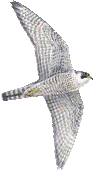## g_angle

VERSION 4.6.4
Wed 13 Nov 2013

### Description

g_angle computes the angle distribution for a number of angles or dihedrals.

With option -ov, you can plot the average angle of a group of angles as a function of time. With the -all option, the first graph is the average and the rest are the individual angles.

With the -of option, g_angle also calculates the fraction of trans dihedrals (only for dihedrals) as function of time, but this is probably only fun for a select few.

With option -oc, a dihedral correlation function is calculated.

It should be noted that the index file must contain atom triplets for angles or atom quadruplets for dihedrals. If this is not the case, the program will crash.

With option -or, a trajectory file is dumped containing cos and sin of selected dihedral angles, which subsequently can be used as input for a principal components analysis using g_covar.

Option -ot plots when transitions occur between dihedral rotamers of multiplicity 3 and -oh records a histogram of the times between such transitions, assuming the input trajectory frames are equally spaced in time.

### Files

optionfilenametypedescription
-f traj.xtc Input Trajectory: xtc trr trj gro g96 pdb cpt
-n angle.ndx Input Index file
-od angdist.xvg Output xvgr/xmgr file
-ov angaver.xvg Output, Opt. xvgr/xmgr file
-of dihfrac.xvg Output, Opt. xvgr/xmgr file
-ot dihtrans.xvg Output, Opt. xvgr/xmgr file
-oh trhisto.xvg Output, Opt. xvgr/xmgr file
-oc dihcorr.xvg Output, Opt. xvgr/xmgr file
-or traj.trr Output, Opt. Trajectory in portable xdr format

### Other options

optiontypedefaultdescription
-[no]h bool no Print help info and quit
-[no]version bool no Print version info and quit
-nice int 19 Set the nicelevel
-b time 0 First frame (ps) to read from trajectory
-e time 0 Last frame (ps) to read from trajectory
-dt time 0 Only use frame when t MOD dt = first time (ps)
-[no]w bool no View output .xvg, .xpm, .eps and .pdb files
-xvg enum xmgrace xvg plot formatting: xmgrace, xmgr or none
-type enum angle Type of angle to analyse: angle, dihedral, improper or ryckaert-bellemans
-[no]all bool no Plot all angles separately in the averages file, in the order of appearance in the index file.
-binwidth real 1 binwidth (degrees) for calculating the distribution
-[no]periodic bool yes Print dihedral angles modulo 360 degrees
-[no]chandler bool no Use Chandler correlation function (N[trans] = 1, N[gauche] = 0) rather than cosine correlation function. Trans is defined as phi < -60 or phi > 60.
-[no]avercorr bool no Average the correlation functions for the individual angles/dihedrals
-acflen int -1 Length of the ACF, default is half the number of frames
-[no]normalize bool yes Normalize ACF
-P enum 0 Order of Legendre polynomial for ACF (0 indicates none): 0, 1, 2 or 3
-fitfn enum none Fit function: none, exp, aexp, exp_exp, vac, exp5, exp7, exp9 or erffit
-ncskip int 0 Skip this many points in the output file of correlation functions
-beginfit real 0 Time where to begin the exponential fit of the correlation function
-endfit real -1 Time where to end the exponential fit of the correlation function, -1 is until the end

### Known problems

• Counting transitions only works for dihedrals with multiplicity 3